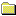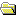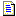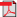Products Download Events Support Videos All Product Families ARM7, ARM9, and Cortex-M3 Products C16x, XC16x, and ST10 Products C251 and 80C251 Products Cx51 and 8051 Products Modified Anytime In the Last 7 Days In the Last 14 Days In the Last 30 Days In the Last 90 Days In the Last 6 Months

Technical Support

On-Line Manuals

Compiler User GuidePrefaceOverview of the CompilerGetting Started with the CompilerCompiler FeaturesCompiler Coding PracticesCompiler Diagnostic MessagesUsing the Inline and Embedded Assemblers of the ARCompiler Command-line OptionsLanguage ExtensionsCompiler-specific FeaturesC and C++ Implementation DetailsWhat is Semihosting?Via File SyntaxSummary Table of GNU Language ExtensionsStandard C Implementation DefinitionStandard C++ Implementation DefinitionC and C++ Compiler Implementation LimitsC++ ISO/IEC standard limitsLimits for integral numbersLimits for floating-point numbers

# <!-- var kmNextPage = ""; var kmPrevPage = "armcc_chr1359125038144.htm"; var kmNextPageTitle = ""; var kmPrevPageTitle = "Limits for integral numbers"; var kmBreadCrumbs = "<a href=\"default.htm\">Home</a> &raquo; <a href=\"armcc_chr1359125037582.htm\">C and C++ Compiler Implementation Limits</a> &raquo; Limits for floating-point numbers"; kmSetupPaging (); kmNavButtons(); //--> Limits for floating-point numbers

## 16.3 Limits for floating-point numbers

This topic describes the characteristics of floating-point numbers.

The following table gives the limits for floating-point numbers. These constants are defined in the float.h include file.

Table 16-3 Floating-point limits

Constant Meaning Value
`FLT_MAX` Maximum value of `float`. 3.40282347e+38F
`FLT_MIN` Minimum normalized positive floating-point number value of `float`. 1.175494351e–38F
`DBL_MAX` Maximum value of `double`. 1.79769313486231571e+308
`DBL_MIN` Minimum normalized positive floating-point number value of `double`. 2.22507385850720138e–308
`LDBL_MAX` Maximum value of `long double`. 1.79769313486231571e+308
`LDBL_MIN` Minimum normalized positive floating-point number value of `long double`. 2.22507385850720138e–308
`FLT_MAX_EXP` Maximum value of base 2 exponent for type `float`. 128
`FLT_MIN_EXP` Minimum value of base 2 exponent for type `float`. –125
`DBL_MAX_EXP` Maximum value of base 2 exponent for type `double`. 1 024
`DBL_MIN_EXP` Minimum value of base 2 exponent for type `double`. –1 021
`LDBL_MAX_EXP` Maximum value of base 2 exponent for type ```long double```. 1 024
`LDBL_MIN_EXP` Minimum value of base 2 exponent for type ```long double```. –1 021
`FLT_MAX_10_EXP` Maximum value of base 10 exponent for type `float`. 38
`FLT_MIN_10_EXP` Minimum value of base 10 exponent for type `float`. –37
`DBL_MAX_10_EXP` Maximum value of base 10 exponent for type `double`. 308
`DBL_MIN_10_EXP` Minimum value of base 10 exponent for type `double`. –307
`LDBL_MAX_10_EXP` Maximum value of base 10 exponent for type ```long double```. 308
`LDBL_MIN_10_EXP` Minimum value of base 10 exponent for type ```long double```. –307
The following table describes other characteristics of floating-point numbers. These constants are also defined in the float.h include file.

Table 16-4 Other floating-point characteristics

Constant Meaning Value
`FLT_RADIX` Base (radix) of the ARM floating-point number representation. 2
`FLT_ROUNDS` Rounding mode for floating-point numbers. (nearest) 1
`FLT_DIG` Decimal digits of precision for `float`. 6
`DBL_DIG` Decimal digits of precision for `double`. 15
`LDBL_DIG` Decimal digits of precision for ```long double```. 15
`FLT_MANT_DIG` Binary digits of precision for type `float`. 24
`DBL_MANT_DIG` Binary digits of precision for type `double`. 53
`LDBL_MANT_DIG` Binary digits of precision for type ```long double```. 53
`FLT_EPSILON` Smallest positive value of x that 1.0 + x != 1.0 for type `float`. 1.19209290e–7F
`DBL_EPSILON` Smallest positive value of x that 1.0 + x != 1.0 for type `double`. 2.2204460492503131e–16
`LDBL_EPSILON` Smallest positive value of x that 1.0 + x != 1.0 for type `long double`. 2.2204460492503131e–16L

### Note

• When a floating-point number is converted to a shorter floating-point number, it is rounded to the nearest representable number.
• Floating-point arithmetic conforms to IEEE 754.
 Non-ConfidentialPDF version ARM DUI0375H Copyright © 2007, 2008, 2011, 2012, 2014-2016 ARM. All rights reserved.##### Important information

This site uses cookies to store information on your computer. By continuing to use our site, you consent to our cookies.